How Cheenta works to ensure student success?
Explore the Back-Story

# Gradient, Divergence and Curl | IIT JAM 2014 | Problem 5Try this problem from IIT JAM 2014 exam. It deals with calculating Gradient of a scalar point function, Divergence and curl of a vector point function.

##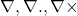Operators | IIT JAM 2014 | Problem 5

If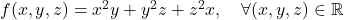and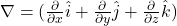then the value of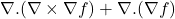at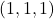••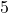•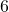•### Key Concepts

Vector Calculus

Scalar Point Function

Answer:IIT JAM 2014 , Problem 5

## Try with Hints

Scalar Point Function : is a function which assigns a point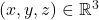to a scalar. Here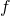is a scalar point function.Gradient of a function :(Divergence of a function () =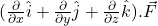Curl of a function () =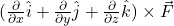Now,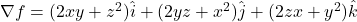Therefore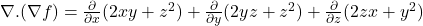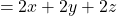Again,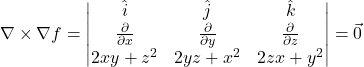Therefore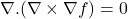then,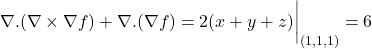## Subscribe to Cheenta at Youtube

Try this problem from IIT JAM 2014 exam. It deals with calculating Gradient of a scalar point function, Divergence and curl of a vector point function.

##Operators | IIT JAM 2014 | Problem 5

Ifandthen the value ofat••••### Key Concepts

Vector Calculus

Scalar Point Function

Answer:IIT JAM 2014 , Problem 5

## Try with Hints

Scalar Point Function : is a function which assigns a pointto a scalar. Hereis a scalar point function.Gradient of a function :(Divergence of a function () =Curl of a function () =Now,ThereforeAgain,Thereforethen,## Subscribe to Cheenta at Youtube

This site uses Akismet to reduce spam. Learn how your comment data is processed.

### Knowledge Partner